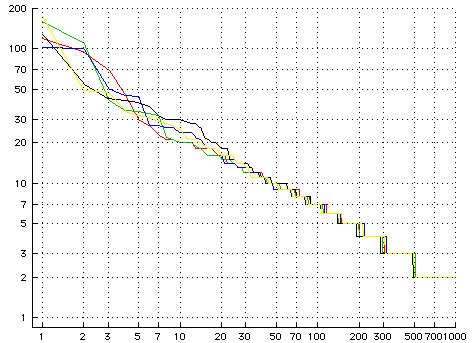# Growing Powerlaw Graphs

I believe the earliest paper that outlines the process that gives rise to a power-law distribution is Herb Simon’s paper from the 1950s that explains that the distribution of words found in human language texts (pdf). He explained them by modeling the process of word selection in those texts by proposing that the next word is selected based on the popularity of the previous words that have been attached to the text.

Barbasi and Albert’s revisit this model (pdf) in the context of growing a network. Their model can be illustrated by this simple function that builds a graph. As we will see the resulting graph will exhibit a power-law distribution in the node connection counts.

``````
(defun make-pgraph (n)
(loop
with g = (make-initial-pgraph n)
finally (return g)
for i from 1 below n
as node1 = (find-prefered-node g)
as node2 = (find-prefered-node g)
``````

This function grows a graph where each node adds two edges to the network. The function proceeds by creating an initial node, who’s two edges are self connected. It then proceeds to add the remaining N-1 nodes with two edges connected to two nodes, node1 and node2. We select which node to connect to via find-prefered-node.

All the debates about what gives rise to power-law distributions arise from arguments about the behavior of the find-prefered-node function. Here’s the one used here. It randomly picks one of the existing edges and then randomly selects the node at one end of that edge or the other.

``````
(defmethod find-prefered-node ((g pgraph))
(let ((e (svref (edges g) (random (edge-count g)))))
(if (eq 0 (random 2))
(left-node e)
(right-node e))))
``````

Here is a graph showing the wealth distribution of the nodes in five graphs grown by this code. Wealth is measured by the number of connections a node manages to aggregate.I don’t see much merit in that function that guides the preferential attachment.

More code below the fold.

This is ANSI standard Common Lisp.

``` (in-package "COMMON-LISP-USER") (defclass pgraph () ((nodes :type simple-vector :initarg :nodes :accessor nodes) (edges :type simple-vector :initarg :edges :accessor edges) (node-count :type fixnum :accessor node-count :initform 0) (edge-count :type fixnum :accessor edge-count :initform 0))) (defclass pgraph-element () ((pgraph :type pgraph :initarg :pgraph :accessor pgraph))) (defclass node (pgraph-element) ((edges :type list :initform () :accessor edges) (edge-count :type fixnum :initform 0 :accessor edge-count))) (defclass edge (pgraph-element) ((left-node :type node :initarg :left-node :accessor left-node) (right-node :type node :initarg :right-node :accessor right-node))) (defmethod add-node-1 ((g pgraph)) (let ((n (make-instance 'node :pgraph g))) (setf (svref (nodes g) (node-count g)) n) (incf (node-count g)) n)) (defmethod make-edge ((g pgraph) (left-node node) (right-node node)) (let ((e (make-instance 'edge :pgraph g :left-node left-node :right-node right-node))) (flet ((add-edge-to-node (n) (push e (edges n)) (incf (edge-count n)))) (add-edge-to-node left-node) (add-edge-to-node right-node)) (setf (svref (edges g) (edge-count g)) e) (incf (edge-count g)) e)) (defmethod add-node ((g pgraph) (node1 node) (node2 node)) (let ((n (add-node-1 g))) (make-edge g n node1) (make-edge g n node2) n)) (defun make-initial-pgraph (n) (let* ((g (make-instance 'pgraph :nodes (make-array n) :edges (make-array (* 2 n)))) (n (add-node-1 g))) (make-edge g n n) (make-edge g n n) g)) (defmethod find-prefered-node ((g pgraph)) (let ((e (svref (edges g) (random (edge-count g))))) (if (eq 0 (random 2)) (left-node e) (right-node e)))) (defun make-pgraph (n) (loop with g = (make-initial-pgraph n) finally (return g) for i from 1 below n as node1 = (find-prefered-node g) as node2 = (find-prefered-node g) do (add-node g node1 node2))) ```

The graphing is done via this package which in turn assumes you are using one other package by the same author and Macintosh Common Lisp.

``` (defmethod graph-pgraph ((g pgraph)) (log-log-graph (sort (map 'list #'edge-count (nodes g)) #'>) "P-Graph")) (defun log-log-graph (data &optional name) (let* (max-x max-y (data-points (loop for tentitive-x from 1 for pt in data as x = (if (consp pt) (first pt) tentitive-x) as y = (if (consp pt) (second pt) pt) collect (list (log x 10) (log y 10)) into collected-data maximize x into mx maximize y into my finally (setf max-x mx max-y my) (return collected-data)))) (if name (graph:graph data-points name) (graph:graph data-points)) (flet ((make-tick-marks (max) (nreverse (loop named accumulate-ticks with tick-marks = () for i = 1 then (* 10 i) do (labels ((finish () (return-from accumulate-ticks tick-marks)) (push-tick (tick) (push (list (log tick 10) (format nil "~D" tick)) tick-marks)) (add-tick (tick) (push-tick tick) (when (>= tick max) (finish)))) (add-tick i) (add-tick (* 2 i)) (add-tick (* 3 i)) (add-tick (* 5 i)) (add-tick (* 7 i))))))) (apply #'graph:x-tick-marks (make-tick-marks max-x)) (apply #'graph:y-tick-marks (make-tick-marks max-y))) (graph:grid-graph))) (defun display-a-few (n m) (graph-pgraph (make-pgraph m)) (loop for i from 1 below n do (graph:graph+ (loop for i from 1 for c in (sort (map 'list #'edge-count (nodes (make-pgraph 1000))) '>) collect (list (log i 10) (log c 10))) "P-Graph"))) ```